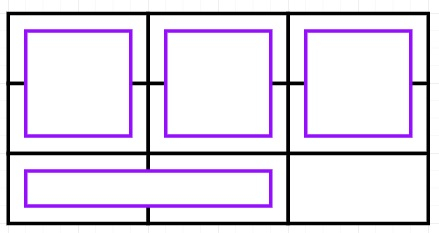# Find the number of rectangles of size 2x1 which can be placed inside a rectangle of size n x m in Python

Suppose we have two values n and m; we have to find the number of rectangles of size 2x1 that can be set inside a rectangle of size n x m. There are few conditions, that we have to consider −

• Any two small rectangles cannot overlap.

• Every small rectangle lies completely inside the bigger one. It is permitted to touch the edges of the bigger rectangle.

So, if the input is liken = 3, m = 3, then the output will be 4

To solve this, we will follow these steps −

• if n mod 2 is same as 0, then

• return(n / 2) * m

• otherwise when m mod 2 is 0, then

• return(m / 2) * n

• return (n * m - 1) / 2

## Example

Let us see the following implementation to get better understanding −

Live Demo

def count_rect(n, m):
if (n % 2 == 0):
return (n / 2) * m
elif (m % 2 == 0):
return (m // 2) * n
return (n * m - 1) // 2
n = 3
m = 3
print(count_rect(n, m))

## Input:

3, 3

## Output

4